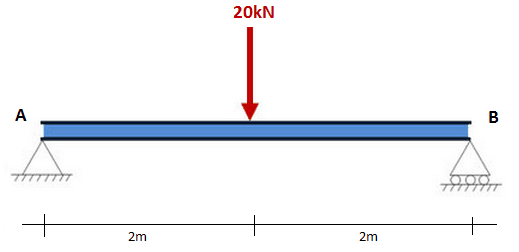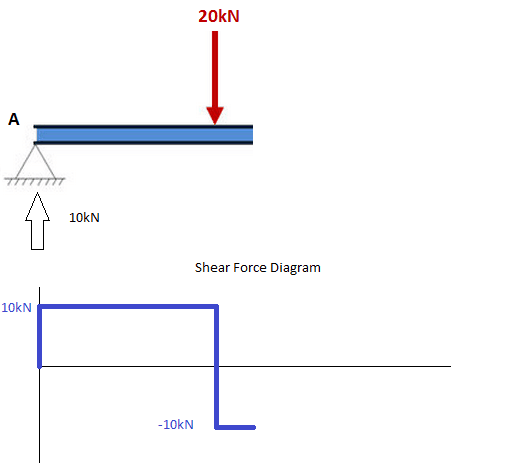The shear force diagram (S.F.D) is drawn to represent the variation of shear force along a beam. The positive shear force is drawn as ordinate above arbitrary reference line and negative shear force below it. The straight lines or curves joining the tips of all such ordinates at salient points from the S.F.D.

The steps to draw a S.F.D are as follows:
If there is an upward force (i.e a support) then the S.F.D will start at this force above the x-axis. If there is downward point load and no support, then the shear force diagram will start as a negative at the value of the point load.
• Move across the beam
As you have come across loads, you simply add (or subtract) these loads from the value you already have, keeping a cumulative total.
It is much easier to understand when considering an example of how to calculate the shear force diagram.
So lets consider the following example to calculate the shear force diagram of a beam:Step 1: After calculating the reactions at A and B, start the shear force diagram at the first value of the force acting on the beam. In this case it is a +10 KN due to the reaction at point A.Step 2: Keep moving across the beam, stopping at every load that acts on the beam. When you get to a load, add to the shear force diagram by the amount of the force. In this case we have come to a negative 20 KN force, so we will minus 20 KN form the existing 10 KN.

10 KN - 20 KN = -10 KNStep 2 (repeated): Moving across the beam again, we come to another force; a positive 10 KN reaction at support B. Again add this +10 KN to the shear force diagram (which is currently at -10 KN) which will bring us to a shear force of 0. Since we are at the end of the beam, we will go no further and we have our final shear force diagram (S.F.D).Special features of S.F.D:
• The area under the S.F.D above the x-axis should equal the area between the x-axis and the S.F.D below the x-axis. i.e the area should sum to zero.
• Any points where the S.F.D cross the x-axis, will be a maximum or minimum bending moment.
• The S.F.D should always equal zero at both ends